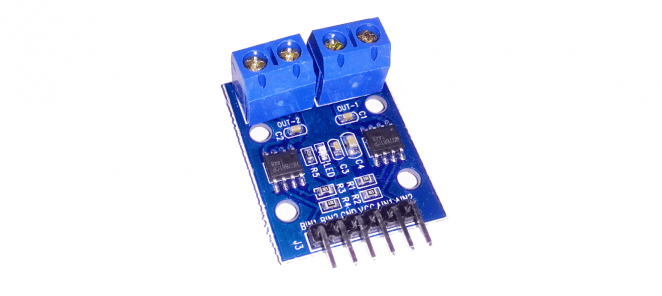# How to use H-bridge HG7881 (HG7881CP) module with external power supply and Arduino UNO

First-time use of H-bridge HG7881 (HG7881CP) module was pretty confusing for me. I didn’t find good (if any?) information about wiring this module up and running it with Arduino. But same time it seems to be quite popular. Here I share how I connected it to an external 12V power supply. You can use any other voltage between ~5V – 12V. Lower voltages might have some problems discussed in this thread.

This module can regulate speed (voltage) and direction (polarity). My example code demonstrates both actions.

## Wiring H-bridge HG7881 and code example

Since this is a 2-channel module, we can operate two different motors with it. To obtain a better overview, I only use one channel (motor) in this wiring schema and example code.

HG7881CP Arduino / Power supply Power supply GND Power supply +12V Arduino digital D8 (motor direction) Arduino digital D9 (motor speed)

Arduino GND must also be connected to the power supply GND terminal. I think it’s a good idea to add also a  resistor between power supply GND and Arduino GND (to limit possible over-current). You can read about this problem from this forum thread.

A closer look at the wiring at HG7881 module side.

Now you can upload example code to Arduino. If everything is wired up correctly motor should start spinning in both directions with a 3-second pause between direction switch. Same time increasing speed from min to max speed (0V to power supply voltage) for both directions. Note that there is inverting of speed value at other direction. This is Arduino sketch file I used.

Increasing speed doesn’t necessarily need to start from 0 or 225. You have to find your own sweet spot where the motor minimum voltage is reached which makes it running.

``````
/**
* H-bridge module HG7881CP/HG7881CP example code
* http://diyprojects.eu/how-to-use-h-bridge-hg7881-with-external-power-supply-and-arduino
*/

/**
* Create variables to be used to run motor A
*/
int motorAPin_A = 8; //Arduino digital 8 is connected to HG7881's A-1A terminal
int motorAPin_B = 9; //Arduino digital 9 is connected to HG7881's A-1B terminal

void setup(){
/**
* When program starts set Arduino pinmode for 8 and 9 digital to be OUTPUT
* so we can use analogWrite to output values from 0 to 255 (0-5V) (PWM)
*/
pinMode(motorAPin_A, OUTPUT); //direction
pinMode(motorAPin_B, OUTPUT); //speed
}

void loop() {
//set motor direction to "X"
analogWrite(motorAPin_A, LOW);

//start motor and increase speed while spinnning to direction "X"
for(int i=0; i<=255; i++){
//motor speed increases while we loop trough
//values from 0 to 255 (0V to power supply max voltage)
analogWrite(motorAPin_B, i);
delay(40);
}

//wait 3 seconds while motor is running full speed
delay( 3000 );

//take 1 second pause, cutting power from motor ( speed pint to 0V )
//so motor can stop (maybe your motor needs more time to spin down)
analogWrite(motorAPin_A, LOW);
analogWrite(motorAPin_B, LOW);
delay(1000);

//now we switch direction to "Y" by setting 5V to
//direction pin A-1A
analogWrite(motorAPin_A, 255);

//start motor and increase speed while spinnning to direction "Y"
for(int i=0; i<=255; i++){
//To speed up like we did in direction "X" this module needs
//inverting of value we set on speed pin.
//So we go trough loop like before, but this time speed value
//decreases from 255 to 0 (via inverting value)
analogWrite(motorAPin_B, invertOurValue( i ) );
delay(40);
}

//wait 3 seconds while motor is running full speed
delay( 3000 );

//take 1 second pause, cutting power from motor ( speed pint to 0V )
analogWrite(motorAPin_A, LOW);
analogWrite(motorAPin_B, LOW);
delay(1000);

}

int invertOurValue(int input) {
return 255 - input;
}
``````

## The result of running this example code

Following video demonstrates how this example code should run the motor. Note that whining at the start- it’s generated by PWM signal and if the voltage is too low, it won’t start. Just whines. Every motor has it’s own minimum operating voltage so they can behave differently.

1. By Pratik

2. By David

• By Janar

• By Dave

3. By Marius

• By Pukkita

• By Janar

4. By ash